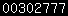Here you find the hyperlink to download the propagation calculation program. After downloading you can run this program and calculate the expected signal to noise ratio for a contact between two stations. The program calculates for line off sight contacts and for troposcatter contacts. Inputs must be given for: distance between the two stations: if input = 0 then an extra question is asked with calculation between two different Maidenhaid locators or E-locators. Input must be given with capital letters. Frequency to work on. Antenna height of both stations. In meter ASL Output power of both stations. In Watt Noise figure of both stations. Information about obstacles with distance and height of the obstacle. Antenna gain; if input=0 then a calculation is done based upon dish antenna with question for antenna diameter. The calculation is done with 50% efficiency. Bandwith used; for regular narrowband contacts use 3000 Hz On frequency's of 24 GHz and above the program corrects propagation loss for extra loss due to humidity. Adding about 0.1 dB for each Km. An update is under construction where the humidity losses can be calculated with different temperatures and humidity. The outputs of the program are expected signal to noise ratio for a line of sight contact and for a troposcatter contact.   When using this program it gives good indication on the chance to make a QSO. Difficult is the situation with anamolous propagation. The way I have been using up to now is to make a contact on for example 10 GHz and measure the received S/N ratio. Then make the calculation based on the stations data for 10 GHz. Take the calculated S/N ratio for a line of sight contact and compare this to the actual S/N ratio. Take the difference , for example 25 dB, and keep this difference for reference. Then make a calculation for 24 GHz based on the data of both 24 GHz stations, and take the calculated S/N ratio for a line of sight contact. Last thing to do is to subtract the reference difference for the 10 GHz contact from the calculated S/N ratio of the 24 GHz line of sight. When this gives positive value's a contact on 24 GHz could be very well possible. This method gives good indications on possible 24 GHz contacts.   Download the program   :(35 kB)